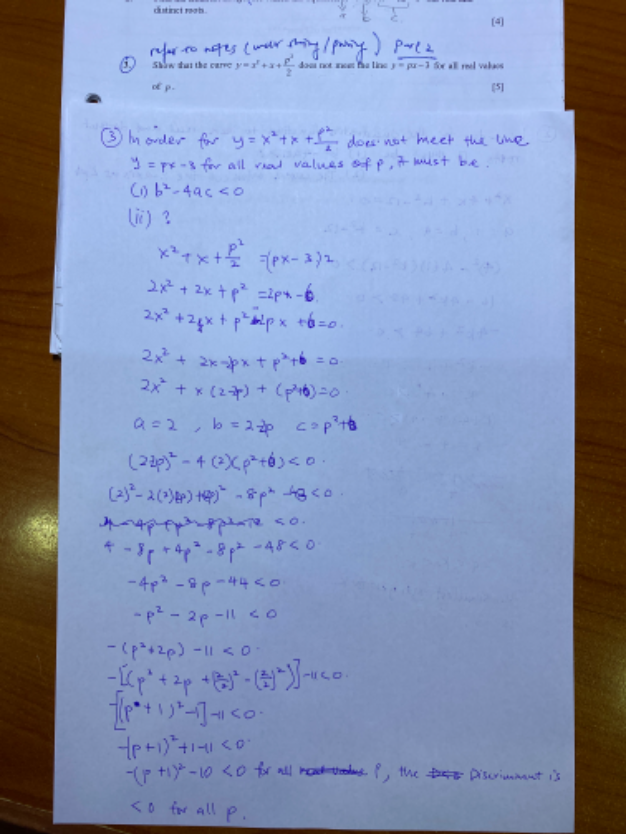# QuestionMay I know what should I filled up on ii) for no real value of p? Is my ending explanation enough to ‘show’ question?

Your solution is somewhat correct, just a bit complex.

For no real roots, only need to prove that D < 0,  of the resulting quadratic equation (same as all the other resulting quadratic equations in the earlier questions)

2 + x + (p2 /2) = px – 3

2 + (1-p)x +  (p2 /2) +3 = 0

a = 1, b = (1-p) ,  c =  (p2 /2) +3

D = b2 – 4ac = (1-p) – 4(1)[(p2 /2) +3]

= 1 -p2 -2p -2p2 -12

= – p -2p -11

= – [ p + 2p + 11 ]

= – [ (p + 2p + 1) – 1 + 11 ]  (complete the square)

= – [(p+1)2  + 10 ]

Since  [(p+1)2 + 10 ] is a positive number for all values of p,  having a negative sign makes  – [(p+1)2  + 10 ] a negative number.

Hence  D = – [(p+1)2  + 10 ] < 0 implies that x 2 + x + (p2 /2) = px – 3 has no real roots for all values of p.